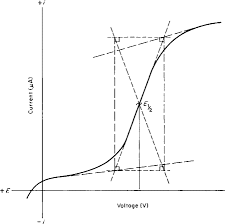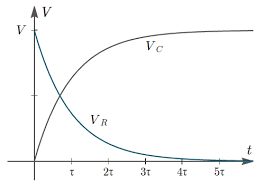## How to Calculate and Solve for Nernst Equation | Corrosion

The nernst equation is illustrated by the image below.To compute for nernst equation, four essential parameters are needed and these parameters are Electrochemical Cell Potential (ΔV°), Number of Electrons (n), Molar Concentration [M1n+] and Molar Concentration [M2n+].

The formula for calculating nernst equation:

ΔV = ΔV° – 0.0592/n log(M1n+/M2n+)

Where:

ΔV = Nernst Equation | Potential
ΔV° = Electrochemical Cell Potential
n = Number of Electrons
M1n+ = Molar Concentration
M2n+ = Molar Concentration

Given an example;
Find the nernst equation when the electrochemical cell potential is 3, the number of electrons is 9, the molar concentration is 6 and the molar concentration is 2.

This implies that;

ΔV° = Electrochemical Cell Potential = 3
n = Number of Electrons = 9
M1n+ = Molar Concentration = 6
M2n+ = Molar Concentration = 2

ΔV = ΔV° – 0.0592/n log(M1n+/M2n+)
ΔV = 3 – (0.0592/9) log(6/2)
ΔV = 3 – (0.00657) log (3)
ΔV = 3 – 0.0065 (0.477)
ΔV = 3 – (0.0031)
ΔV = 2.99

Therefore, the nernst equation is 2.99 V.

## How to Calculate and Solve for Nernst Equation | Corrosion

The nernst equation is illustrated by the image below.To compute for nernst equation, seven essential parameters are needed and these parameters are Electrochemical Cell Potential (ΔV°), Gas Constant (R), Temperature (T), Number of Electrons (n), Faraday’s Constant (F), Molar Concentration [M1n+] and Molar Concentration [M2n+].

The formula for caluclating nernst equation:

ΔV = ΔV° – RT/nF In(M1n+/M2n+)

Where:

ΔV = Nernst Equation | Potential
ΔV° = Electrochemical Cell Potential
R = Gas Constant
T = Temperature
n = Number of Electrons
M1n+ = Molar Concentration
M2n+ = Molar Concentration

Let’s solve an example;
Find the nernst equation when the electrochemical cell potential is 8, the gas constant is 2, the temperature is 5, the number of electrons is 3, the faraday’s constant is 9, the molar concentration is 10 and the molar concentration is 4.

This implies that;

ΔV° = Electrochemical Cell Potential = 8
R = Gas Constant = 2
T = Temperature = 5
n = Number of Electrons = 3
F = Faraday’s Constant = 9
M1n+ = Molar Concentration = 10
M2n+ = Molar Concentration = 4

ΔV = ΔV° – RT/nF In(M1n+/M2n+)
ΔV = 8 – ((2)(5)/(3)(9)) In(10/4)
ΔV = 8 – (10/27) In (2.5)
ΔV = 8 – 0.37 (0.91)
ΔV = 8 – (0.339)
ΔV = 7.66

Therefore, the nernest equation is 7.66 V.